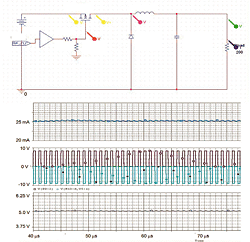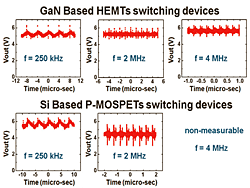Ph.D. student Chandler Seo with adviser K. KimFigure 10: Schematic diagram of DC-DC converter and simulation results: output current Iout, voltage at the gate and drain, and output voltage signal Vout versus time.

A dc-dc converter transfers energy between two dc circuits operating at different voltage and current levels. It is possible to change the output voltage and current level by varying the duty ratio (if the pulse has duration DT where T is the period and D is the duty ratio) of the power switching system. In a previous study, we improved the contact resistance of GaN metal semiconductor
transistors using the selective area growth (SAG) technique facilitated by plasma assisted molecular beam epitaxy (PAMBE). Nonalloyed high electron mobility transistors (HEMT)s were successfully fabricated with a peak drain current and maximum transconductance of 573 mA/mm and 60 mS/mm, respectively. In the present work, we design a dc-dc converter and attempt to demonstrate it with GaN-based power devices.Figure 11: Output voltage (Vout) of DC-DC converters utilizing GaN-based HEMTs and Si-based P-MOSFETs with varying input frequency from 250 kHz to 4 MHz.

The direct switching converter for dc voltage to dc current is shown in Figure 10. We designed a buck converter where the output is lower than the input. First, we tried the switching test at 250 kHz with GaN- based HETMs. The duty ratio was 60 % and with changes in the gate signal, the voltage between the drain and source changed with matching gate input. We raised the frequency to 4 MHz and tested the dc-dc converter. The output voltage was calculated using the following equation: Vout = D1 × Vin, where duty ratio D1 and input voltage Vin were 0.6 and 8.4 V, respectively. The expected output voltage was 5.04 V. Figure 11 shows the output voltage for various input frequencies. Using the HEMTs, the output signals were almost constant and output voltage ripple was ± 0.5 V. When the input frequency increased, the ripple increased only slightly. On the other hand, output signals with Si-based P-MOSFETs showed similar voltage output ripple at 250 kHz, but with increasing input frequencies up to 2 MHz, the output ripple increased up to ±1.5 V and at higher frequencies, e.g., 4 MHz, the signals were dominated by noise and resonance. As a result, in the latter case, the converter could not operate normally and a reliable signal could not be measured. In summary, the dc-dc converters built with GaN-based HETMs showed less noise and better performance in the high-frequency region than those with Si-based P-MOSFETs.

This work is supported by the Grainger Center for Electric Machinery and Electromechanics.